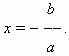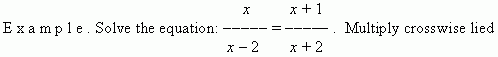# Linear equations in one unknown

An equation of the shape: ax + b = 0, where a and b – the known numbers, x – an unknown value, is called a linear equation in one unknown. To solve this equation means to find the numerical value of x , at which this equation becomes an identity.

If a is not equal to zero ( a 0 ),  then a solution  ( root )  has the shape:If a = 0 , then the two cases are possible:

1 . b = 0, then 0 · x + 0 = 0 . Here x can be any number ( check this ! ).

2 . b ≠ 0, then 0 · x + b = 0 . There is no solution ( check this also ).expressions: x 2 + 2 x = x 2 2 x +  x – 2 . Transfer all terms to the

left-hand side of the equation. After reducing all similar terms we’ll

receive: 3 x + 2 = 0, hence x = – 2 / 3 .